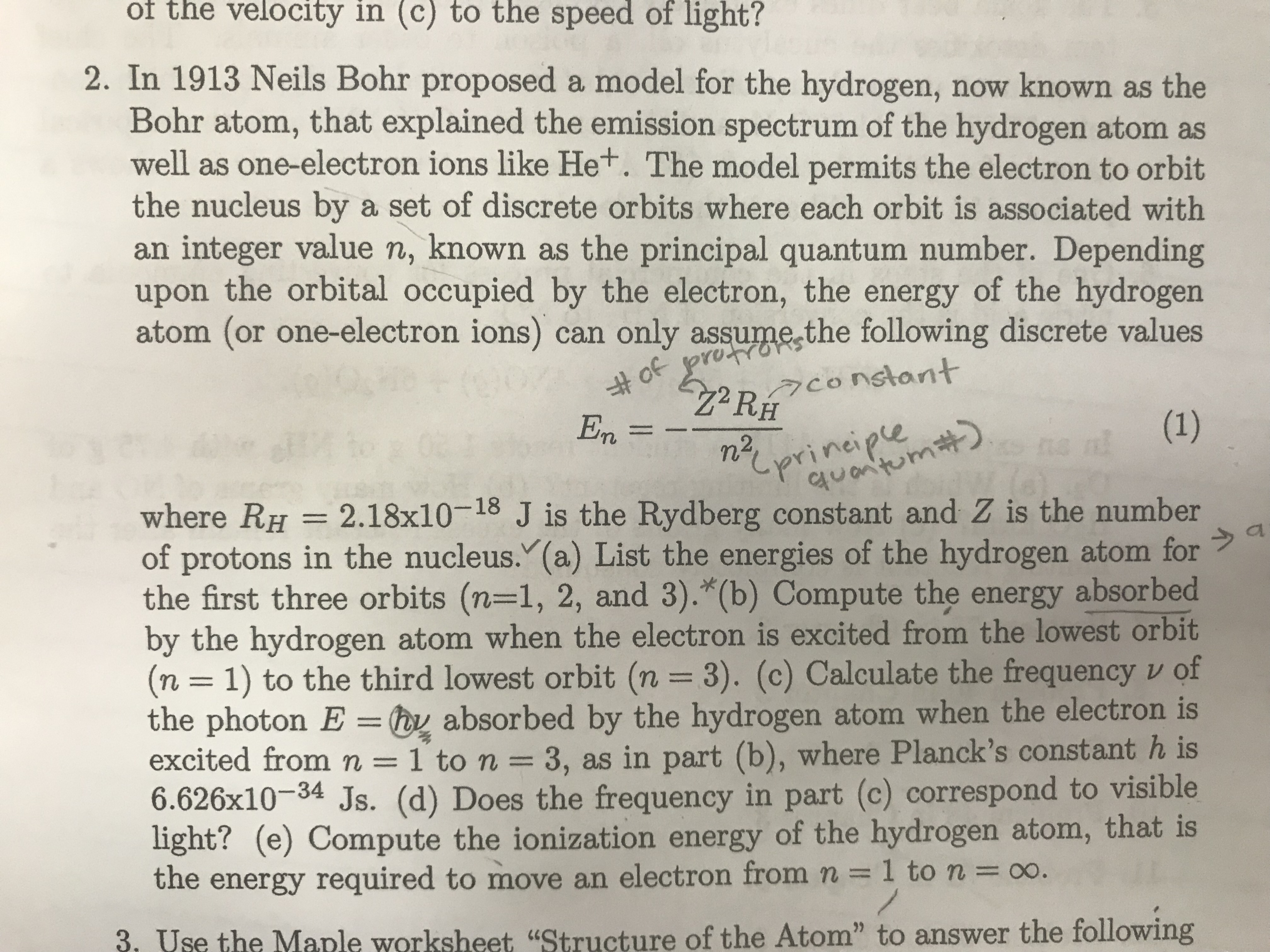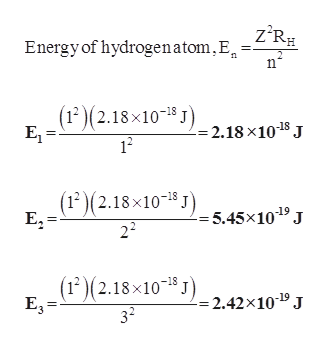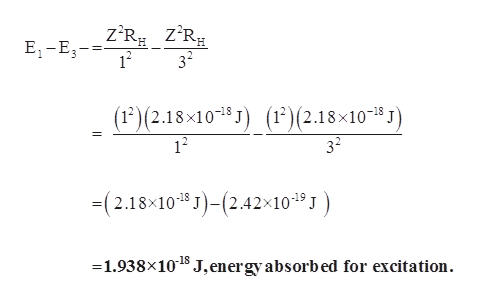# of the velocity in (c) to the speed of light?2. In 1913 Neils Bohr proposed a model for the hydrogen, now known as theBohr atom, that explained the emission spectrum of the hydrogen atom aswell as one-electron ions like Het. The model permits the electron to orbitthe nucleus by a set of discrete orbits where each orbit is associated withan integer value n, known as the principal quantum number. Dependingupon the orbital occupied by the electron, the energy of the hydrogenatom (or one-electron ions) can only assume the following discrete values1terd 30 tFaconstantEnn2prineiple2 S1where RH 2.18x10-18 J is the Rydberg constant and Z is the numberof protons in the nucleus.(a) List the energies of the hydrogen atom forthe first three orbits (n-1, 2, and 3).*(b) Compute the energy absorbedby the hydrogen atom when the electron is excited from the lowest orbit(n 1) to the third lowest orbit (n 3). (c) Calculate the frequency v ofthe photon E = y absorbed by the hydrogen atom when the electron isexcited fromn=1 to n =3, as in part (b), where Planck's constant h is6.626x10-34 Js. (d) Does the frequency in part (c) correspond to visiblelight? (e) Compute the ionization energy of the hydrogen atom, that is(T)1the energy required to move an electron from n= 1 to n = oo.3. Use the Maple worksheet "Structure of the Atom" to answer the following

Question
3 viewshelp_outlineImage Transcriptioncloseof the velocity in (c) to the speed of light? 2. In 1913 Neils Bohr proposed a model for the hydrogen, now known as the Bohr atom, that explained the emission spectrum of the hydrogen atom as well as one-electron ions like Het. The model permits the electron to orbit the nucleus by a set of discrete orbits where each orbit is associated with an integer value n, known as the principal quantum number. Depending upon the orbital occupied by the electron, the energy of the hydrogen atom (or one-electron ions) can only assume the following discrete values 1terd 30 tF aconstant En n2 prineiple 2 S1 where RH 2.18x10-18 J is the Rydberg constant and Z is the number of protons in the nucleus.(a) List the energies of the hydrogen atom for the first three orbits (n-1, 2, and 3).*(b) Compute the energy absorbed by the hydrogen atom when the electron is excited from the lowest orbit (n 1) to the third lowest orbit (n 3). (c) Calculate the frequency v of the photon E = y absorbed by the hydrogen atom when the electron is excited fromn=1 to n =3, as in part (b), where Planck's constant h is 6.626x10-34 Js. (d) Does the frequency in part (c) correspond to visible light? (e) Compute the ionization energy of the hydrogen atom, that is (T) 1 the energy required to move an electron from n= 1 to n = oo. 3. Use the Maple worksheet "Structure of the Atom" to answer the following fullscreen
check_circle

Step 1

Hey, since there are multiple subparts present, we will answer first three subparts. If you want any specific subparts to be answered then please submit that question only or specify the subpart number in your message.

Step 2

(a)

Energy for the hydrogen atom for the first three orbits (E1, E2 and E3) can be calculated as follows,help_outlineImage TranscriptioncloseEnergy of hydrogenatom,E =Z°R# n (12)(2.18x10 -2.18 x10 8 J E1 12 (12)(2.18x10 J5,45x10 J 22 (12)(2.18x10 J2.42x10* J E3 -18 32 fullscreen
Step 3

(b)

The energy absorbed by hydrogen atom when the electron is excited f...help_outlineImage TranscriptioncloseE1-E R z'R 12 32 (1)(2.18x101 J (1?)(2.18x1038 J) 12 32 -(2.18x10 J)(2.42x104 1.938x10 J, energyabsorbed for excitation. fullscreen

### Want to see the full answer?

See Solution

#### Want to see this answer and more?

Solutions are written by subject experts who are available 24/7. Questions are typically answered within 1 hour.*

See Solution
*Response times may vary by subject and question.
Tagged in

### Atomic structure